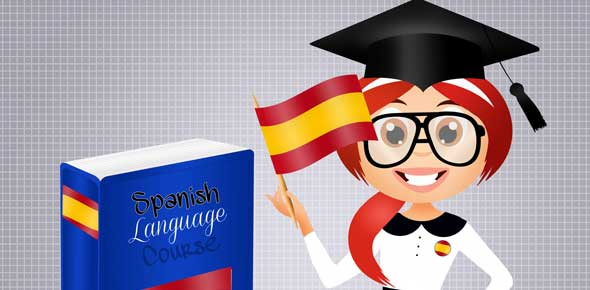# Spanish Prepositions Vocab Test

14 Questions | Total Attempts: 448SettingsRelated Topics
• 1.
Write the translation  entre = ___?___
• 2.
Write the translation  delante (de) = ___?___
• 3.
Write the translation  detrás (de) = ___?___
• 4.
Write the translation  al lado (de) = ___?___
• 5.
Write the translation  encima (de) = ___?___
• 6.
Write the translation  debajo (de) = ___?___
• 7.
Write the translation  enfrente (de) = ___?___
• 8.
Write the translation  between = ___?___
• 9.
Write the translation  in front (of) = ___?___
• 10.
Write the translation  behind (of) = ___?___
• 11.
Write the translation  next to = ___?___
• 12.
Write the translation  on top (of) = ___?___
• 13.
Write the translation  under = ___?___
• 14.
Write the translation  opposite (of) = ___?___